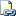# CREATE TYPE (Transact-SQL)Transact-SQL 语法约定

## 语法

``````-- User-defined Data Type syntax
CREATE TYPE [ schema_name. ] type_name
{
[
FROM base_type
[ ( precision [ , scale ] ) ]
[ NULL | NOT NULL ]
]
| EXTERNAL NAME assembly_name [ .class_name ]
| AS TABLE ( { <column_definition> | <computed_column_definition> [ ,... n ] }
[ <table_constraint> ] [ ,... n ]
[ <table_index> ] [ ,... n ] } )

} [ ; ]

<column_definition> ::=
column_name <data_type>
[ COLLATE collation_name ]
[ NULL | NOT NULL ]
[
DEFAULT constant_expression ]
| [ IDENTITY [ ( seed ,increment ) ]
]
[ ROWGUIDCOL ] [ <column_constraint> [ ...n ] ]

<data type> ::=
[ type_schema_name . ] type_name
[ ( precision [ , scale ] | max |
[ { CONTENT | DOCUMENT } ] xml_schema_collection ) ]

<column_constraint> ::=
{     { PRIMARY KEY | UNIQUE }
[ CLUSTERED | NONCLUSTERED ]
[
WITH ( <index_option> [ ,...n ] )
]
| CHECK ( logical_expression )
}

<computed_column_definition> ::=

column_name AS computed_column_expression
[ PERSISTED [ NOT NULL ] ]
[
{ PRIMARY KEY | UNIQUE }
[ CLUSTERED | NONCLUSTERED ]
[
WITH ( <index_option> [ ,...n ] )
]
| CHECK ( logical_expression )
]

<table_constraint> ::=
{
{ PRIMARY KEY | UNIQUE }
[ CLUSTERED | NONCLUSTERED ]
( column [ ASC | DESC ] [ ,...n ] )
[
WITH ( <index_option> [ ,...n ] )
]
| CHECK ( logical_expression )
}

<index_option> ::=
{
IGNORE_DUP_KEY = { ON | OFF }
}

< table_index > ::=
INDEX constraint_name
[ CLUSTERED | NONCLUSTERED ] (column [ ASC | DESC ] [ ,... n ] )} }
``````
``````-- User-defined Memory Optimized Table Types syntax
CREATE TYPE [schema_name. ] type_name
AS TABLE ( { <column_definition> [ ,... n ] }
| [ <table_constraint> ] [ ,... n ]
| [ <table_index> ] [ ,... n ] } )
[ WITH ( <table_option> [ ,... n ] ) ]
[ ; ]

<column_definition> ::=
column_name <data_type>
[ COLLATE collation_name ] [ NULL | NOT NULL ]    [
[ IDENTITY [ (1 , 1) ]
]
[ <column_constraint> [, ... n ] ]    [ <column_index> ]

<data type> ::=
[type_schema_name . ] type_name [ ( precision [ , scale ]) ]

<column_constraint> ::=
{ PRIMARY KEY { NONCLUSTERED HASH WITH ( BUCKET_COUNT = bucket_count )
| NONCLUSTERED }
}

< table_constraint > ::=
{ PRIMARY KEY { NONCLUSTERED HASH (column [ ,... n ] )
WITH ( BUCKET_COUNT = bucket_count )
| NONCLUSTERED ( column [ ASC | DESC ] [ ,... n ] )
}
}

<column_index> ::=
INDEX index_name
{ [ NONCLUSTERED ] HASH WITH ( BUCKET_COUNT = bucket_count )
| NONCLUSTERED
}

< table_index > ::=
INDEX constraint_name
{ [ NONCLUSTERED ] HASH (column [ ,... n ] ) WITH (BUCKET_COUNT = bucket_count)
|  [ NONCLUSTERED ]  ( column [ ASC | DESC ] [ ,... n ] )
}

<table_option> ::=
{
[ MEMORY_OPTIMIZED = { ON | OFF } ]
}
``````

## 参数

schema_name

type_name

base_type
SQL Server 提供的数据类型，别名数据类型以此类型为基础。 base_type 为 sysname，无默认值，并且可以是下列值之一：

bigint
binary( n )
bit
char( n )

date
datetime
datetime2
datetimeoffset

decimal
float

int

money
nchar( n )
ntext
numeric

nvarchar(n | max)
real
smalldatetime
smallint

smallmoney
sql_variant
text
time

tinyint
uniqueidentifier
varbinary(n | max)
varchar(n | max)

base_type 还可以是映射到这些系统数据类型之一的任何数据类型同义词。

scale

NULL | NOT NULL

assembly_name

EXTERNAL_NAME 在包含数据库中不可用。

[.class_name]

<column_definition>

<数据类型>

<column_constraint>

<computed_column_definition>

<table_constraint>

<index_option>

`INDEX *index_name* [ CLUSTERED | NONCLUSTERED ] (*column_name* [ ASC | DESC ] [ ,... *n* ] )`

INDEX

MEMORY_OPTIMIZED

BUCKET_COUNT

HASH

## 备注

• 类可以具有重载方法，但只能从托管代码内而不能从 Transact-SQL 调用这些方法。

• 如果 assembly_name 是 SAFE 或 EXTERNAL_ACCESS，则必须将所有静态成员声明为 const 或 readonly 。

## 示例

### A. 基于 varchar 数据类型创建别名类型

``````CREATE TYPE SSN
FROM varchar(11) NOT NULL ;
``````

### B. 创建用户定义类型

``````CREATE ASSEMBLY utf8string
AUTHORIZATION [dbi]
FROM 0x4D... ;
GO
CREATE TYPE Utf8String
EXTERNAL NAME utf8string.[Microsoft.Samples.SqlServer.utf8string] ;
GO
``````

### C. 创建用户定义表类型

``````CREATE TYPE LocationTableType AS TABLE
( LocationName VARCHAR(50)
, CostRate INT );
GO
``````

### D. 创建包含主键和索引的用户定义表类型

``````CREATE TYPE InventoryItem AS TABLE
(
[Name] NVARCHAR(50) NOT NULL,
SupplierId BIGINT NOT NULL,
Price DECIMAL (18, 4) NULL,
PRIMARY KEY (
Name
),
INDEX IX_InventoryItem_Price (
Price
)
)
GO
``````